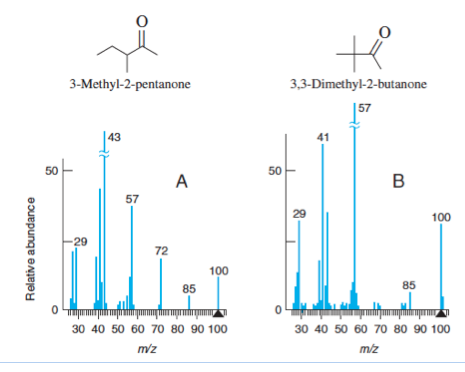# Problem: Spectra A and B belong to the isomers of C6H12O below. Explain how you can tell which isomer goes with each spectrum.

###### Problem Details

Spectra A and B belong to the isomers of C6H12O below. Explain how you can tell which isomer goes with each spectrum.Frequently Asked Questions

What scientific concept do you need to know in order to solve this problem?

Our tutors have indicated that to solve this problem you will need to apply the Mass Spectrometry concept. You can view video lessons to learn Mass Spectrometry. Or if you need more Mass Spectrometry practice, you can also practice Mass Spectrometry practice problems.

What is the difficulty of this problem?

Our tutors rated the difficulty ofSpectra A and B belong to the isomers of C6H12O below. Expla...as low difficulty.

How long does this problem take to solve?

Our expert Analytical Chemistry tutor, Jules took 2 minutes and 29 seconds to solve this problem. You can follow their steps in the video explanation above.